## The Riemann Hypothesis: FAQ and resources

Riemann Hypothesis quotes

Riemann Hypothesis FAQ

• What is the Riemann Hypothesis?

The Riemann Hypothesis is a mathematical conjecture, first proposed in 1859 and still unproven as of 2015. It's arguably the most famous of all unresolved mathematical problems, sometimes referred to as "the Holy Grail of mathematics". Although it's related to many areas of mathematics, it's usually thought of as concerning the distribution of prime numbers.

• Who was Riemann?

Bernhard Riemann (full name Georg Friedrich Bernhard Riemann, 1826–1866) was a shy, humble German mathematician who made significant contributions to several areas of mathematics including analysis and differential geometry. He only wrote one paper on number theory, but it was this which contained the statement of his Hypothesis, and so it's easily one of the most important number theory papers ever published. As well as this, his work on differential geometry paved the way for the mathematical foundations of Einstein's general theory of relativity.• How is it connected to prime numbers?

To really answer that question would require quite a lot of higher mathematics, so I can only provide a sketch here, but further resources to help you explore this matter can be found below.

The prime numbers appear throughout the sequence of counting numbers but fail to display any obvious pattern. They show a tendency to thin out, though, and the "average rate" at which they thin out is described by the Prime Number Theorem. That was first proposed at the end of the 1700s, but not proved for another hundred years. In order to prove the PNT, mathematicians needed to study a mathematical object known as the Riemann zeta function. The zeta function was introduced in Riemann's 1859 paper and shown to (in some sense) control the fluctuations of the prime numbers around their "average" behaviour. The zeta function operates on a two-dimensional "number plane" called the complex plane, and associated with it is an infinite set of points known as its "nontrivial zeros" (commonly known as the "zeta zeros" or "Riemann zeros"). The positions of these zeros on the complex plane can be related to an infinite set of wave-like entities which collectively govern the fluctuation of the primes. All of the zeros Riemann was able to calculate lay on a vertical line, and he hypothesised that all of the zeta function's (nontrivial) zeros lie on this "critical line". That is the Riemann Hypothesis. From his writings, it seems that he didn't realise how important this casual assertion would become – he simply stated that he believed it to be true, but that it wasn't directly relevant to his investigations, and moved on.

Riemann was able to prove certain things about the zeta zeros, including that they all must lie in a vertical strip one unit wide (the "critical strip"), centred on the "critical line" mentioned above. In the 1800s, it was shown that the Prime Number Theorem would be true if the zeta zeros could all be shown to lie properly inside the critical strip, that is, not on its edges. In 1896, the mathematicians Hadamard and de la Vallée Poussin proved this almost simultaneously, thereby proving the PNT.

Further narrowing the strip in which the zeta zeros are all known to lie would lead to more precise information on the distribution of prime numbers. The ultimate achievement would be to reduce this strip to its central line (the "critical line"), as narrow as it's possible to get. If this can be done, the RH is proved, and we would know that the prime numbers are as "well behaved" as possible. If the RH is false, there will be zeta zeros which do not lie on the critical line, and the wave-like entities associated with these would result in huge fluctuations in the distribution of primes, thereby disrupting a certain "balance" within the number system which the mathematical community almost universally hopes and believes to be in effect.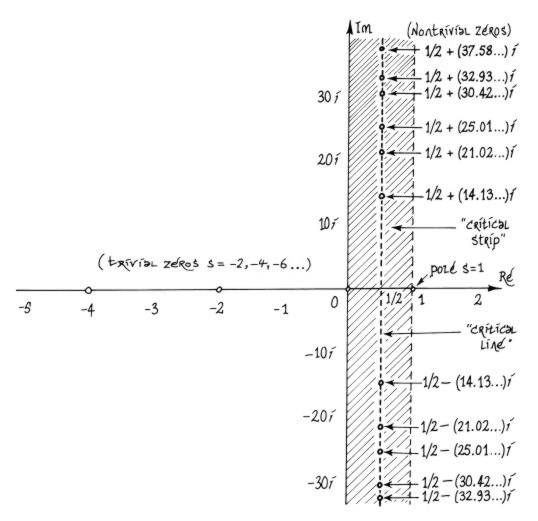• What other areas of mathematics does it relate to?

Just about every area of mathematics can be somehow related to the Riemann Hypothesis. This isn't so surprising when you consider the foundational role the prime numbers play in the number system which underlies all of mathematics. The RH has been "reformulated" as (i.e., shown to be mathematically equivalent to) mathematical conjectures in a staggering diversity of areas. I've collected some of these reformulations here.

• What's this about a \$1,000,000 prize?

The non-profit Clay Mathematics Institute was founded in 1998, and in 2000 announced its seven "Millenium Prize Problems", offering a million-dollar prize for each. Naturally, the Riemann Hypothesis was one of these problems. This led to a huge burst of popular interest in the problem, but as its proof was already the ultimate prize for mathematicians, the million dollars was unlikely to make much difference to them. Needless to say, the prize is still unclaimed.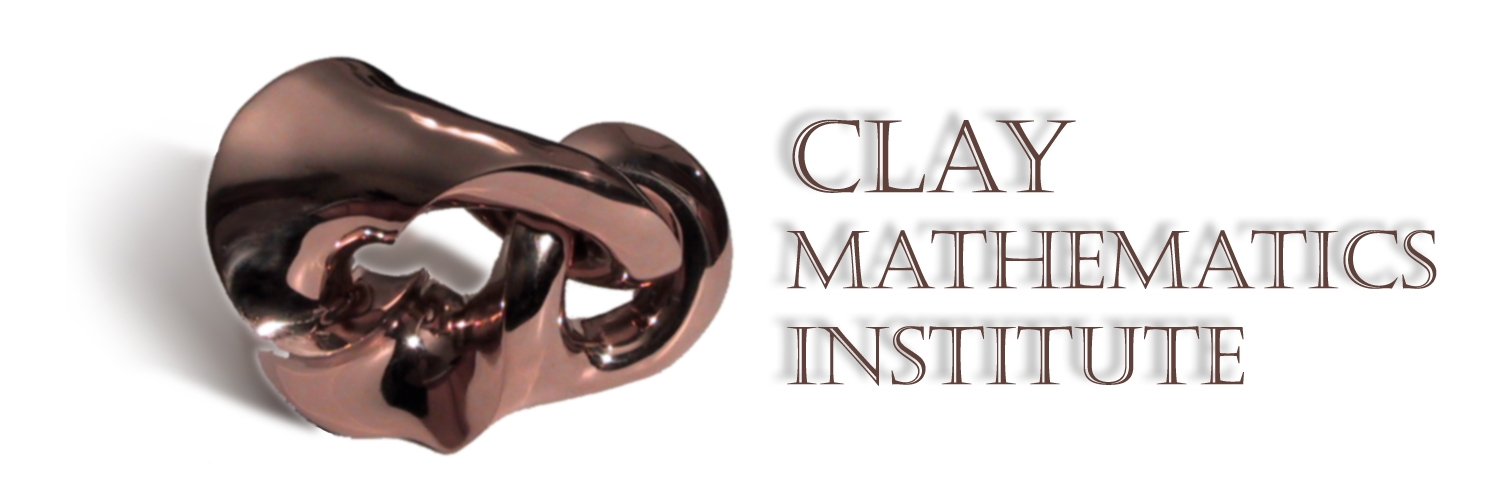• Why is it important?

Is it? Why is anything important? Most peoples' lives would be entirely unaffected by the RH being proved (or disproved). However, within mathematics it's hugely important. Because of the foundational role the prime numbers play in the number system, the RH can be related to many diverse areas of mathematics. Hundreds of theorems exist whose statements begin by assuming the RH is true. Consequently, if the RH is disproved, all of these theorems will collapse, and if it's proved, they will stand. The RH being false would be a disaster for mathematics as we currently understand it.

Also, the fact that over 150 years of dedicated effort have failed to produce a proof means that mathematicians are talking about things like "a gaping hole in our understanding", or a vast chasm between where we are now, mathematically, and where we need to be to prove the RH. This suggests that in order to prove the Hypothesis, some major new ideas are needed, ideas which might fundamentally alter our understanding of the number system. So the pursuit of a proof of the RH is important in that sense.

It should be added that it's the various "generalisations" (see below) of the RH whose proof or disproof would have a truly major impact on mathematics.

Explaining the importance of the Riemann Hypothesis for mathematics is almost as difficult as explaining what it is, so you might want to look at various other peoples' attempts here, here, here and here.

• Are there any proposed proofs circulating?

Yes, there are quite a few. Some are clearly to be taken more seriously than others. The mathematician Louis de Branges, who proved a major result called the Bieberbach Conjecture in 1985, has put forward several proposed proofs, the most recent at the end of 2014. He's the best known of all the "proof" authors, some of whom are professional mathematicians, the majority being amateurs.

I've been archiving all proposed proofs and disproofs here for some years now, including false alarms, April Fool proofs, comedy proofs and at least one "theological" argument for the RH!

• Who is considered to be in the running to prove the RH?

It depends who you ask. Louis de Branges is a serious mathematician with a formidable track record, but his particular approach to the RH doesn't seem to have won many followers in the mathematical community. Alain Connes' approach involving noncommutative geometry seems to be the one most people involved see as potentially fruitful. Christopher Deninger's name also comes up sometimes. Karl Sabbagh's book Dr. Riemann's Zeros (2002), while rather lacking in terms of explaining the mathematics of the RH, contains a good overview of the human side of the story, so that would be a good starting point for answering this question.

• What's considered to be the most likely approach to succeed in proving the RH?

In the late 1990s, it seemed like Alain Connes' work in noncommutative geometry was the way forward, with some promising papers published. But that research seems to have reached an impasse in the last decade or so.

It depends who you ask! Any mathematician who thinks they're on their way to a proof would consider their approach to be the most likely to succeed. And then there's the possibility that one or more heavyweight mathematicians are working on the problem secretively (as Andrew Wiles did with Fermat's Last Theorem) using an approach none of us know about, on the verge of completing a proof. It's believed that Paul Cohen (1934–2007) and Atle Selberg (1917–2007) were both "secretly" working on the Riemann Hypothesis up until their deaths.

Oxford University number theorist Roger Heath-Brown has said that "no longer [are] just analytic number theorists involved, but all mathematicians know about the problem, and many realise that they may have useful insights to offer. As far as I can see, a solution is as likely to come from a probabilist, geometer or mathematical physicist, as from a number theorist."

• Does anyone believe it to be false?

In 1962, the accomplished Cambridge number theorist John Littlewood (best known for his collaborations with G.H. Hardy) published a short piece in which he bluntly stated that he believed it to be false, that there's no evidence at all and no imaginable reason why it should be true. It could be argued that this was just bitterness borne from his inability to prove it himself (his doctoral supervisor had rather cruelly set him the problem at a time when it wasn't as well known). In 2008, Aleksandar Ivić published some reasons why he was skeptical about the truth of the RH.

• Could its truth or falsehood turn out to be undecidable?

We can't rule this out. Mathematician and computer scientist Gregory Chaitin has published some thoughts on how Gödel's Incompleteness Theorems (concerning the existence of undecidable proposition within axiomatic systems) might be relevant to the RH and how it could possibly be undecidable (see here).

• Are there any books on the RH for the layperson? Has anyone written a "Riemann Hypothesis for Dummies" or "Riemann Hypothesis simplified"?

There are several. In 2003, due to the burst of interest generated by the CMI's \$1,000,000 prize offer, three popular mathematics books were published on the RH. John Derbyshire's Prime Obsession is the most mathematically detailed, but would be hard to follow without degree-level mathematics. Karl Sabbagh's Dr. Riemann's Zeros was light on the mathematics, but provides a detailed portrait of many of the mathematicians involved, focussing on the "human angle". Marcus du Sautoy's The Music of the Primes was somewhere between these two, covering both the mathematical and cultural angles. Comparative reviews of these books can be found here, here and here. A few years later, Dan Rockmore's Stalking the Riemann Hypothesis appeared, which is quite technical in some places, but very readable in others.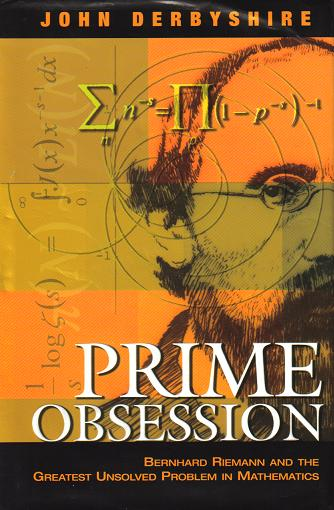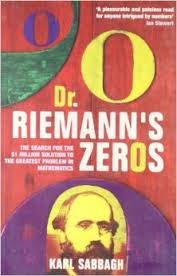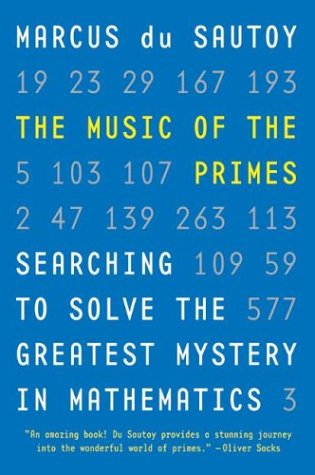Having been curating this website for some years, I wanted to created a book which truly communicated the mathematics of the Riemann Hypothesis (rather than just giving the reader a feeling of what's involved) and which my non-mathematical friends could read. This led to me working with an illustrator to develop a new, primarily visual approach to some otherwise inaccessible mathematical concepts, and the original book idea eventually gave rise to a trilogy of books. The Secrets of Creation trilogy first explores the distribution of prime numbers, leading to a detailed account of Riemann's zeta function and Hypothesis in Volume 2. The final volume considers the connection with quantum physics and its philosophical implications.

• I think I have a proof of the RH! What do I do now?

Stay calm. There's a very good chance you're mistaken. After all, this problem has been around for over 150 years and many of the best mathematical minds on the planet have been grappling with it for most of that time. Because of my web-presence, I get sent proposed proofs by amateurs from time to time, and post them here. One recurring concern that their authors express is that someone will steal the idea from them before they get the \$1 million prize. That shouldn't be a concern. Create a simple website and post your work there – that's sufficient evidence of original authorship. Send me a link and I'll post it on my page of proposed RH proofs. You can use the sci.math newsgroup or the Prime Pages email list to attract attention to your work.

Unfortunately, though, most mathematicians just haven't got time to read proposed proofs of the RH when they are almost 100% sure the author is somehow mistaken. As someone once said, "It's easier to prove the Riemann Hypothesis than it is to get someone to read your proof!" Your best hope is that an interested postgrad or mathematician with some spare time will scan through your work, fail to find any problems with it, and forward it on to someone higher up the ladder of mathematical prestige.

For more on how to get your work out there and any concerns you might have about it being stolen, read this.

• I've heard something about a connection with quantum physics – what's that about?

To understand this requires a familiarity with quantum physics, chaos theory and the Riemann zeta function, so the best I can do here is to give a very sketchy outline. Part of Riemann's work on the distribution of primes showed that the "prime counting function" can be understood in terms of a set of wave-like mathematical objects. As with waves in physics, these have wavelengths and frequencies. There's an infinite number of them and their frequencies collectively make up what's called a "spectrum". In the early 1980s, the physicist Michael Berry noticed that this spectrum corresponds remarkably closely to the spectrum associated with a type of physical oscillating system. The truth or falsehood of the Riemann Hypothesis can then be linked to physical properties of the system in question. This opens up the possibility that the discovery of (the possible existence of) a certain physical system could lead to a proof of the RH.

Although it's very common to find mathematical structures reflected in physical reality (this is the basis of modern physics), this is a very strange reversal of that situation, where a physical structure is mirrored in mathematical reality. A very specific class of "quantum chaological" oscillators appears to somehow underlie the distribution of prime numbers (and thereby the system of counting numbers). No one knows what this means, and it's the strangest thing I'm aware of in my experience of reality! This is all patiently explained (without any prerequisite maths or physics) in the final volume of my Secrets of Creation trilogy.

• Isn't there a connection with cryptography? Would a proof compromise the security of Internet communications and financial transactions?

The RSA algorithm, commonly used in cryptography, involves the use of large prime numbers and exploits the fact that determining the prime factors of a large composite number is much more laborious than multiplying the factors together in the first place. I've explained in a bit more detail here.

A proof of the Riemann Hypothesis wouldn't, in itself, compromise the RSA algorithm (or others based on number theory). However, the "big new idea(s)" which everyone expects to be needed for a proof of the RH might lead to breakthroughs in the efficient factorising of integers, and that would be a problem for cryptography. These issues are explored in some detail here, here and here.

• What are the Extended Riemann Hypothesis, Generalised RH, Grand RH?

These are also unproved mathematical conjectures and are "generalisations" of the Riemann Hypothesis. That is, the RH in its familiar form can be understood as a special case of each of these. If any of them were proved true, the RH would automatically follow.

Recall that the Riemann Hypothesis, as usually formulated, concerns the zeros of the Riemann zeta function. It turns out that there are many types of zeta functions in mathematics, Riemann's just being a particularly significant one. Among the ever-expanding pantheon of zeta functions we find "Dedekind zeta functions of algebraic number fields". The familiar system of rational numbers (consisting of all ratios of integers – positive, negative and zero) are one instance of an algebraic number field, and the Dedekind zeta function for the rationals turns out to be the same as the Riemann zeta function. The Extended Riemann Hypothesis states that all (nontrivial) zeros of all Dedekind zeta functions lie on the "critical line", so clearly if that's true then all the Riemann zeros lie on the critical line and the RH must be true.

The Generalised Riemann Hypothesis concerns all Dirichlet L-functions, of which the Riemann zeta function is a single example, similarly requiring their zeros to lie on the critical line. The Grand Riemann Hypothesis generalises not only the familiar RH but also the Generalised RH, as it concerns all automorphic L-functions, which include all Dirichlet L-functions.

Riemann Hypothesis quotes

" Hilbert included the problem of proving the Riemann hypothesis in his list of the most important unsolved problems which confronted mathematics in 1900, and the attempt to solve this problem has occupied the best efforts of many of the best mathematicians of the twentieth century. It is now unquestionably the most celebrated problem in mathematics and it continues to attract the attention of the best mathematicians, not only because it has gone unsolved for so long but also because it appears tantalizingly vulnerable and because its solution would probably bring to light new techniques of far reaching importance."

H.M. Edwards, from Riemann's Zeta Function (1974), p.6

"Right now, when we tackle problems without knowing the truth of the Riemann hypothesis, it's as if we have a screwdriver. But when we have it, it'll be more like a bulldozer."

P. Sarnak, from "Prime Time" by E. Klarreich (New Scientist, 11/11/2000)

"The consequences [of the Riemann Hypothesis] are fantastic: the distribution of primes, these elementary objects of arithmetic. And to have tools to study the distribution of these of objects."

H. Iwaniec, quoted in K. Sabbagh's Dr. Riemann's Zeros (Atlantic, 2002), p.30

"If [the Riemann Hypothesis is] not true, then the world is a very different place. The whole structure of integers and prime numbers would be very different to what we could imagine. In a way, it would be more interesting if it were false, but it would be a disaster because we've built so much round assuming its truth."

P. Sarnak, quoted in K. Sabbagh's Dr. Riemann's Zeros (Atlantic, 2002), p.30

"If there are lots of zeros off the line – and there might be – the whole picture is just horrible, horrible, very ugly. It's an Occam's razor sort of thing, you either have absolutely beautiful behaviour of prime numbers, they behave just like you want them to behave, or else it's really bad."

S. Gonek, quoted in Dr. Riemann's Zeros (Atlantic, 2002), p.112

"The Riemann Hypothesis is the most basic connection between addition and multiplication that there is, so I think of it in the simplest terms as something really basic that we don't understand about the link between addition and multiplication."

B. Conrey, quoted in Dr. Riemann's Zeros (Atlantic, 2002), p.160

"[The Riemann Hypothesis] is probably the most basic problem in mathematics, in the sense that it is the intertwining of addition and multiplication. It's a gaping hole in our understanding..."

A. Connes, quoted in Dr. Riemann's Zeros (Atlantic, 2002), p.208

Riemann Hypothesis resources

WolframMathwold: notes on the Riemann Hypothesis

C. Caldwell's basic introduction to the Riemann Hypothesis

K. Spiliopoulos', Introduction to the Riemann Hypothesis

G. Pugh's excellent "The Riemann Hypothesis in a Nutshell", including a Z(t) plotting applet

J. Brian Conrey, "The Riemann Hypothesis", Notices of the AMS (March 2003) – a very nice, comprehensive introduction to the RH

P. Borwein, S. Choi, B. Rooney and A. Weirathmueller, The Riemann Hypothesis: For the afficionado and virtuoso alike (eBook, 2006)

WWN notes on the Riemann Hypothesis (part of a work-in-progress)

Z. Rudnick, "Number theoretic background" (proceedings of a summer school in Bologna, August 2001)
This covers all the number theory necessary for a basic understanding of the Riemann Hypothesis, which is covered in its final section.

Riemann's original eight-page paper
PDF, English translation     other formats

"Riemann wrote only one article on the theory of numbers, published in 1859. This paper radically redrew the landscape of the subject. The specific approach to the distribution of prime numbers he developed, both simple and revolutionary, consists of appealing to Cauchy's theory of holomorphic functions, which at that time was a relatively recent discovery."
G. Tenenbaum and M. Mendès France, from The Prime Numbers and Their Distribution (AMS, 2000)

"The Riemann Hypothesis and its generalisations", part of a work-in-progress, see also the subsections:

J. Baez, This Week's Finds in Mathematical Physics week 217 includes very helpful discussion of the Riemann Hypothesis, Extended Riemann Hypothesis, Grand Riemann Hypothesis, Weil Conjectures, Langlands Programme, the functional equations of zeta and L-functions, modularity of theta functions, etc.

The Clay Mathematics Institute offers \$1,000,000 for a proof of the Riemann Hypothesis

an extremely thorough mathematical description of the Riemann Hypothesis (with historical background, etc.) provided by Enrico Bombieri for the purposes of this competition

video recording of an introductory lecture by J. Vaaler on the RH (one of the Clay Foundation's "Millenium Lectures")

K. Sabbagh, Dr. Riemann's Zeros: The Search for the \$1 Million Solution to the Greatest Problem in Mathematics (Atlantic Books, 2002)

Two more books of a similar nature followed in 2003:

J. Derbyshire, Prime Obsession: Bernhard Riemann and the Greatest Unsolved Problem in Mathematics, (JHP, 2003)

Marcus du Sautoy, The Music of the Primes: Searching to Solve the Greatest Mystery in Mathematics (HaperCollins, 2003)

Here is K. Leutwyler's comparitive review of all three books from Scientific American.

Here is another, by D. Lim, from The Village Voice.

...and another by J.C. Alexander

some proposed proofs and disproofs of the Riemann Hypothesis (some more serious than others!)

J.E. Littlewood's brief argument as to why he believes the Riemann Hypothesis to be false.

Set theorist and mathematical philosopher Gregory Chaitin discusses the possibility that the RH might be undecidable, i.e., there can be no proof.

a popular exposition on the Riemann Hypothesis which appeared in New Scientist (11/11/00)

"The Mark of Zeta": Ivars Peterson's introductory essay on RH and Riemann's zeta function

"The Return of Zeta": sequel article by Ivars Peterson on links between the RH, random matrix theory and quantum chaos

K. Sabbagh, "Beautiful Maths", Prospect (January 2002)

B. Schechter, "143-year-old problem still has mathematicians guessing" (a fairly good New York Times article on zeta functions conference at the Courant Institute, 07/2002)

ZetaGrid: Verification of the Riemann Hypothesis (a project coordinated by S. Wedeniwski of IBM Deutschland, completed 2005)

"Today, we have better resources to verify or falsify Riemann's hypothesis. First the high-speed computers, then the networks have increased the capacity of calculations. Now we want to go one step further by bundling up the resources into a grid network. Therefore, I invite all interested people to participate in the calculation of the zeros of the Riemann zeta function for a new record."

S. Wedeniwski, "Computations connected with the verification of the Riemann Hypothesis" (useful overview with history and references)

A.R. Booker, "Turing and the Riemann Hypothesis", Notices of the AMS 53 (2006) 1208–1211

Critical Strip Explorer v0.67, a wonderful applet produced by Raymond Manzoni for this site – explore the behaviour of the Riemann zeta function in and around the critical strip in a highly visual, interactive way. The resulting images are quite astonishing!

Freeman Dyson's suggested approach to proving the Riemann Hypothesis using quasi-crystals (from his 2009 AMS lecture)

D. Schumayer and D.A.W. Hutchinson, "Physics of the Riemann hypothesis", Rev. Mod. Phys. 83 (2011) 307–330

[abstract:] "Physicists become acquainted with special functions early in their studies. Consider our perennial model, the harmonic oscillator, for which we need Hermite functions, or the Laguerre functions in quantum mechanics. Here we choose a particular number theoretical function, the Riemann zeta function and examine its influence in the realm of physics and also how physics may be suggestive for the resolution of one of mathematics' most famous unconfirmed conjectures, the Riemann Hypothesis. Does physics hold an essential key to the solution for this more than hundred-year-old problem? In this work we examine numerous models from different branches of physics, from classical mechanics to statistical physics, where this function plays an integral role. We also see how this function is related to quantum chaos and how its pole-structure encodes when particles can undergo Bose–Einstein condensation at low temperature. Throughout these examinations we highlight how physics can perhaps shed light on the Riemann Hypothesis. Naturally, our aim could not be to be comprehensive, rather we focus on the major models and aim to give an informed starting point for the interested Reader."

H. Montgomery, A. Nikeghbali and M.T. Rassias, eds., Exploring the Riemann Zeta Function (Springer 2017)

W. Dittrich, Reassessing Riemann's paper on the number of primes less than a given magnitude (Springer, 2018)

number theory and physics archive      prime numbers: FAQ and research
mystery      new      search      home